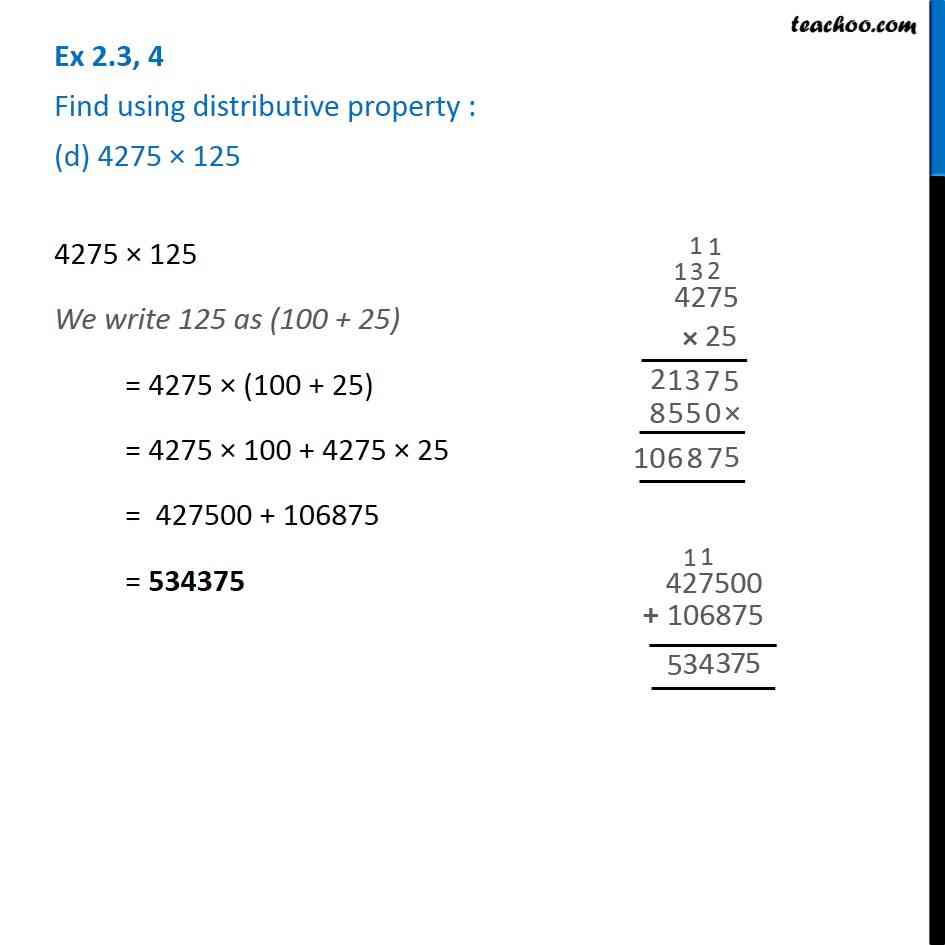1. Chapter 2 Class 6 Whole Numbers
2. Serial order wise
3. Ex 2.3

Transcript

Ex 2.3, 4 Find using distributive property : (d) 4275 × 125 4275 × 125 We write 125 as (100 + 25) = 4275 × (100 + 25) = 4275 × 100 + 4275 × 25 = 427500 + 106875 = 534375